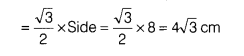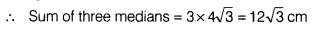# If each side of an equilateral triangle is 8cm,find sum of three medians of this triangle

If each side of an equilateral triangle is 8 cm, then find sum of three medians of this triangle.

Given, side of equilateral triangle = 8cm
∴ Median (or altitude) of an equilateral triangleWe know that three medians are equal of equilateral triangle.